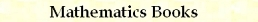Complex Metric C/C++ Macros
by Stanislav Sýkora, Extra Byte, Via R.Sanzio 22C, Castano Primo, Italy 20022
in Stan's Library, Ed.S.Sykora, Vol.II. First release February 15, 2007.
Permalink via DOI:  10.3247/SL2Soft07.002
 PREVIOUS | NEXT | Code snippets | Software Books and Links Programming Section of Stan' LIBRARY | Stan's HUB
 This document is a part of a collection of macros. For introductory remarks, see the first part (General Purpose Macros). For a review of macro-syntax rules and related tips and tricks, see Writing Macros. Naturally enough, the arguments of complex metric macros are assumed to be complex numbers. As explained in the article on Basic Metric Macros, however, the macros listed here can be used as templates for similar ones, involving different entities belonging to different matric sets (such as vectors, matrices or functions). All you have to do is replace the character combination _C in all their names (or in the whole file) by another one, say _X and provide a new definition of the new distance macro mDist_X.
 Distance definition Code: #define mNorm_C(a) sqrt(mReal(a)*mReal(a)+mImag(a)*mImag(a)) #define mDist_C(a,b) mNorm_C((b)-(a)) Arguments: a and b stand for complex numbers or complex-valued expressions. The definition assumes that the function sqrt(x) has been already declaed and that one has pre-defined the elementary macros mReal(a) and mImag(a) for extracting the real and imaginary parts of a complex number. mNorm_C(a) and mDist_C(a,b) These macros encapsulate the formula for the norm (modulus) of a complex number and the corresponding formula for the distance between two complex numbers.
 [Minimum] distance from a set of elements Code: #define mDistTo_C2(x,a,b) mMin(mDist_C(x,a),mDist_C(x,b)) #define mDistTo_C3(x,a,b,c) mMin(mDist_C(x,a),mDistTo_C2(x,b,c)) #define mDistTo_C4(x,a,b,c,d) mMin(mDistTo_C2(x,a,b),mDistTo_C2(x,c,d)) #define mDistTo_C5(x,a,b,c,d,e) mMin(mDistTo_C2(x,a,b),mDistTo_C3(x,c,d,e)) #define mDistTo_C6(x,a,b,c,d,e,f) mMin(mDistTo_C3(x,a,b,c),mDistTo_C3(x,d,e,f)) Arguments: x and a through f are subject to the same requirements as the arguments of the macro mDist_C discussed above. In particular, they may be expressions. The macros rely on the distance-definition macro mDist_C and on the relational macro mMin. mDistTo_C2(x,a,b), mDistTo_C3(x,a,b,c), mDistTo_C4(x,a,b,c,d), mDistTo_C5(x,a,b,c,d,e), mDistTo_C6(x,a,b,c,d,e,f) These macros return the shortest distance between x and the set of elements {a,b,...}.
 Maximum distance from the elements of a set Code: #define mMaxDistTo_C2(x,a,b) mMax(mDist_C(x,a),mDist_C(x,b)) #define mMaxDistTo_C3(x,a,b,c) mMax(mDist_C(x,a),mMaxDistTo_C2(x,b,c)) #define mMaxDistTo_C4(x,a,b,c,d) mMax(mMaxDistTo_C2(x,a,b),mMaxDistTo_C2(x,c,d)) #define mMaxDistTo_C5(x,a,b,c,d,e) mMax(mMaxDistTo_C2(x,a,b),mMaxDistTo_C3(x,c,d,e)) #define mMaxDistTo_C6(x,a,b,c,d,e,f) mMax(mMaxDistTo_C3(x,a,b,c),mMaxDistTo_C3(x,d,e,f)) Arguments: x and a through f are subject to the same requirements as the arguments of the macro mDist_C discussed above. In particular, they may be expressions. The macros rely on the distance-definition macro mDist_C and on the relational macro mMax. mMaxDistTo_C(x,a,b), mMaxDistTo_C2(x,a,b), mMaxDistTo_C3(x,a,b,c), mMaxDistTo_C4(x,a,b,c,d), mMaxDistTo_C5(x,a,b,c,d,e), mMaxDistTo_C6(x,a,b,c,d,e,f) These macros return the largest distance between x and any element of the set {a,b,...}.
 Value of the nearest set element Code: #define mNearest_C2(x,a,b) (mIsLT(mDist_C(x,a),mDist_C(x,b)) ? (a) : (b)) #define mNearest_C3(x,a,b,c) mNearest_C2(x,a,mNearest_C2(x,b,c)) #define mNearest_C4(x,a,b,c,d) mNearest_C2(x,mNearest_C2(x,a,b),mNearest_C2(x,c,d)) #define mNearest_C5(x,a,b,c,d,e) mNearest_C2(x,mNearest_C2(x,a,b),mNearest_C3(x,c,d,e)) #define mNearest_C6(x,a,b,c,d,e,f) mNearest_C2(x,mNearest_C3(x,a,b,c),mNearest_C3(x,d,e,f)) Arguments: x and a through f are subject to the same requirements as the arguments of the macro mDist_C discussed above. In particular, they may be expressions. The macros rely on the distance-definition macro mDist_C and on the relational macro mIsLT. mNearest_C2(x,a,b), mNearest_C3(x,a,b,c), mNearest_C4(x,a,b,c,d), mNearest_C5(x,a,b,c,d,e), mNearest_C6(x,a,b,c,d,e,f) These macros return the value of the element of the set {a,b,...} which is nearest to x.
 Value of the most remote set element Code: #define mRemotest_C2(x,a,b) (mIsGT(mDist_C(x,a),mDist_C(x,b)) ? (a) : (b)) #define mRemotest_C3(x,a,b,c) mRemotest_C2(x,a,mRemotest_C2(x,b,c)) #define mRemotest_C4(x,a,b,c,d) mRemotest_C2(x,mRemotest_C2(x,a,b),mRemotest_C2(x,c,d)) #define mRemotest_C5(x,a,b,c,d,e) mRemotest_C2(x,mRemotest_C2(x,a,b),mRemotest_C3(x,c,d,e)) #define mRemotest_C6(x,a,b,c,d,e,f) \      mRemotest_C2(x,mRemotest_C3(x,a,b,c),mRemotest_C3(x,d,e,f)) Arguments: x and a through f are subject to the same requirements as the arguments of the macro mDist_C discussed above. In particular, they may be expressions. The macros rely on the distance-definition macro mDist_C and on the relational macro mIsGT. mRemotest_C2(x,a,b), mRemotest_C3(x,a,b,c), mRemotest_C4(x,a,b,c,d), mRemotest_C5(x,a,b,c,d,e), mRemotest_C6(x,a,b,c,d,e,f) These macros return the value of the element of the set {a,b,...} which is most remote from x.
 Maximum and minimum separations between set elements Code: #define mMaxSep_C2(a,b) mDist_C(a,b) #define mMaxSep_C3(a,b,c) mMax(mMaxDistTo_C2(a,b,c),mMaxSep_C2(b,c)) #define mMaxSep_C4(a,b,c,d) mMax(mMaxDistTo_C3(a,b,c,d),mMaxSep_C3(b,c,d)) #define mMaxSep_C5(a,b,c,d,e) mMax(mMaxDistTo_C4(a,b,c,d,e),mMaxSep_C4(b,c,d,e)) #define mMaxSep_C6(a,b,c,d,e,f) mMax(mMaxDistTo_C5(a,b,c,d,e,f),mMaxSep_C5(b,c,d,e,f)) #define mMinSep_C2(a,b) mDist_C(a,b) #define mMinSep_C3(a,b,c) mMin(mDistTo_C2(a,b,c),mMinSep_C2(b,c)) #define mMinSep_C4(a,b,c,d) mMin(mDistTo_C3(a,b,c,d),mMinSep_C3(b,c,d)) #define mMinSep_C5(a,b,c,d,e) mMin(mDistTo_C4(a,b,c,d,e),mMinSep_C4(b,c,d,e)) #define mMinSep_C6(a,b,c,d,e,f) mMin(mDistTo_C5(a,b,c,d,e,f),mMinSep_C5(b,c,d,e,f)) Arguments: x and a - f are subject to the same requirements as the arguments of the macro mDist_C discussed above. In particular, they may be expressions. The macros rely on the distance-definition macro mDist_C and on the relational macros mMax and mMin. mMaxSep_C2(a,b) mMaxSep_C3(a,b,c) mMaxSep_C4(a,b,c,d) mMaxSep_C5(a,b,c,d,e) mMaxSep_C6(a,b,c,d,e,f) These macros return the maximum distance between any two elements of the set {a,b,...}. In some contexts, this quantity is known as the diameter of the set. mMinSep_C2(a,b) mMinSep_C3(a,b,c) mMinSep_C4(a,b,c,d) mMinSep_C5(a,b,c,d,e) mMinSep_C6(a,b,c,d,e,f) These macros return the minimum distance between any two elements of the set {a,b,...}.TOP | PREVIOUS | Code snippets | Software Books and Links Programming Section of Stan' LIBRARY | Stan's HUB
 Copyright ©2007 Stanislav Sýkora    DOI: 10.3247/SL2Soft07.002 Designed by Stan Sýkora